# RD Sharma Solutions Class 11 Geometric Progressions Exercise 20.5

In this section, we shall discuss some important properties of geometric progressions and geometric series. Experts have solved the exercise wise problems using simple techniques to help students improve their accuracy in solving problems. Solutions here are explained using a few examples, for students to understand the concepts clearly and to boost their confidence level. Students who aim to score high marks in the board exams can refer to RD Sharma Class 11 Maths Solutions pdf, which can be downloaded easily from the links given below.

## Download the pdf of RD Sharma Solutions for Class 11 Maths Exercise 20.5 Chapter 20 – Geometric Progression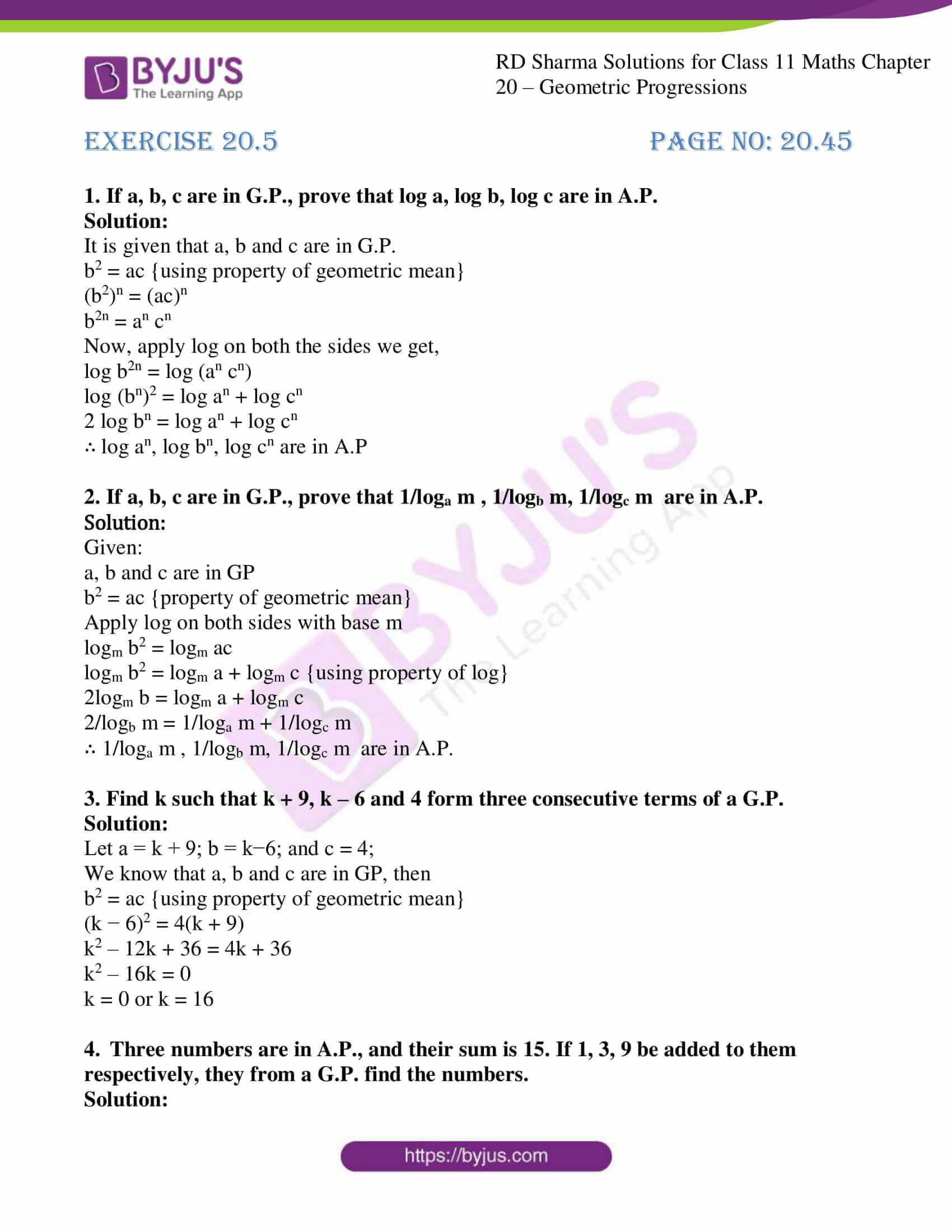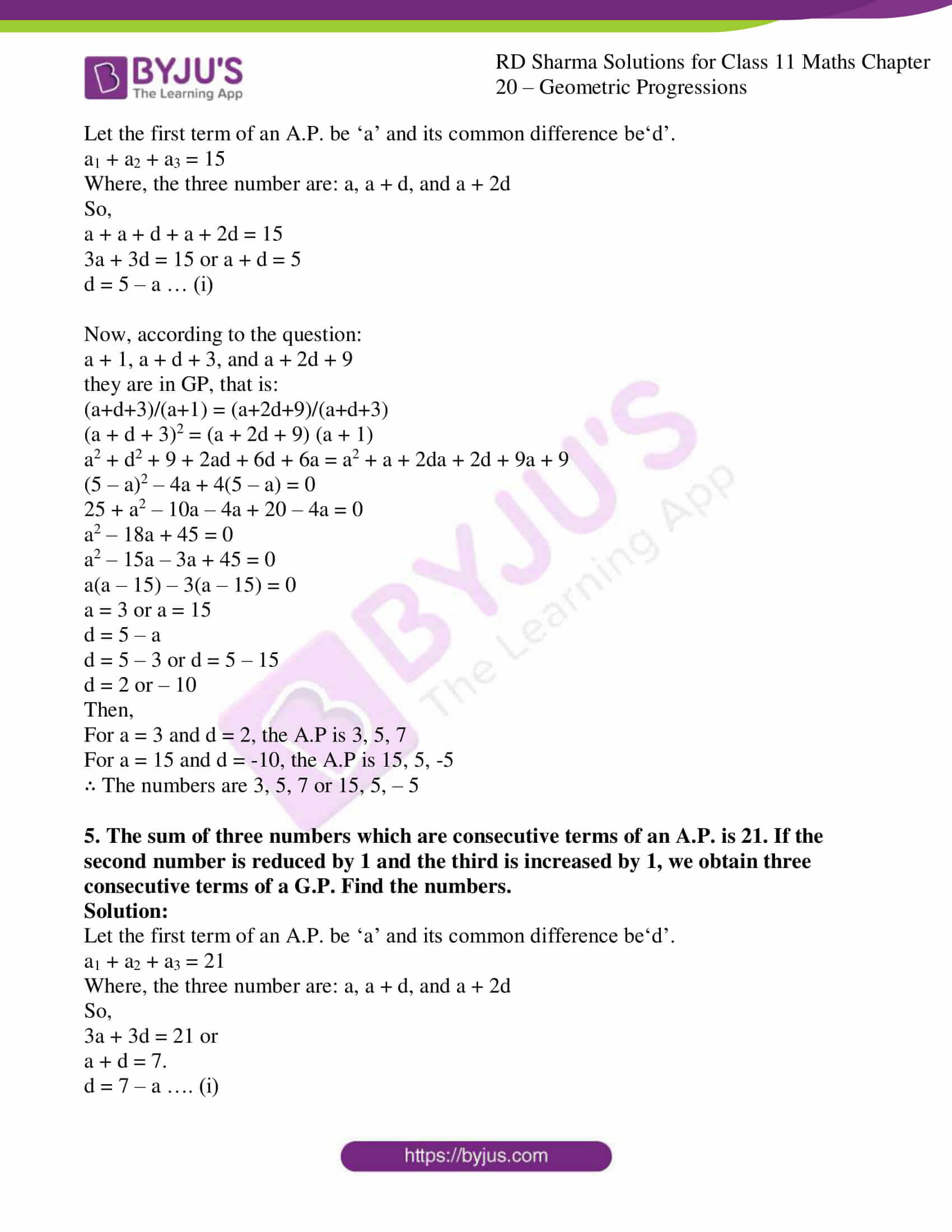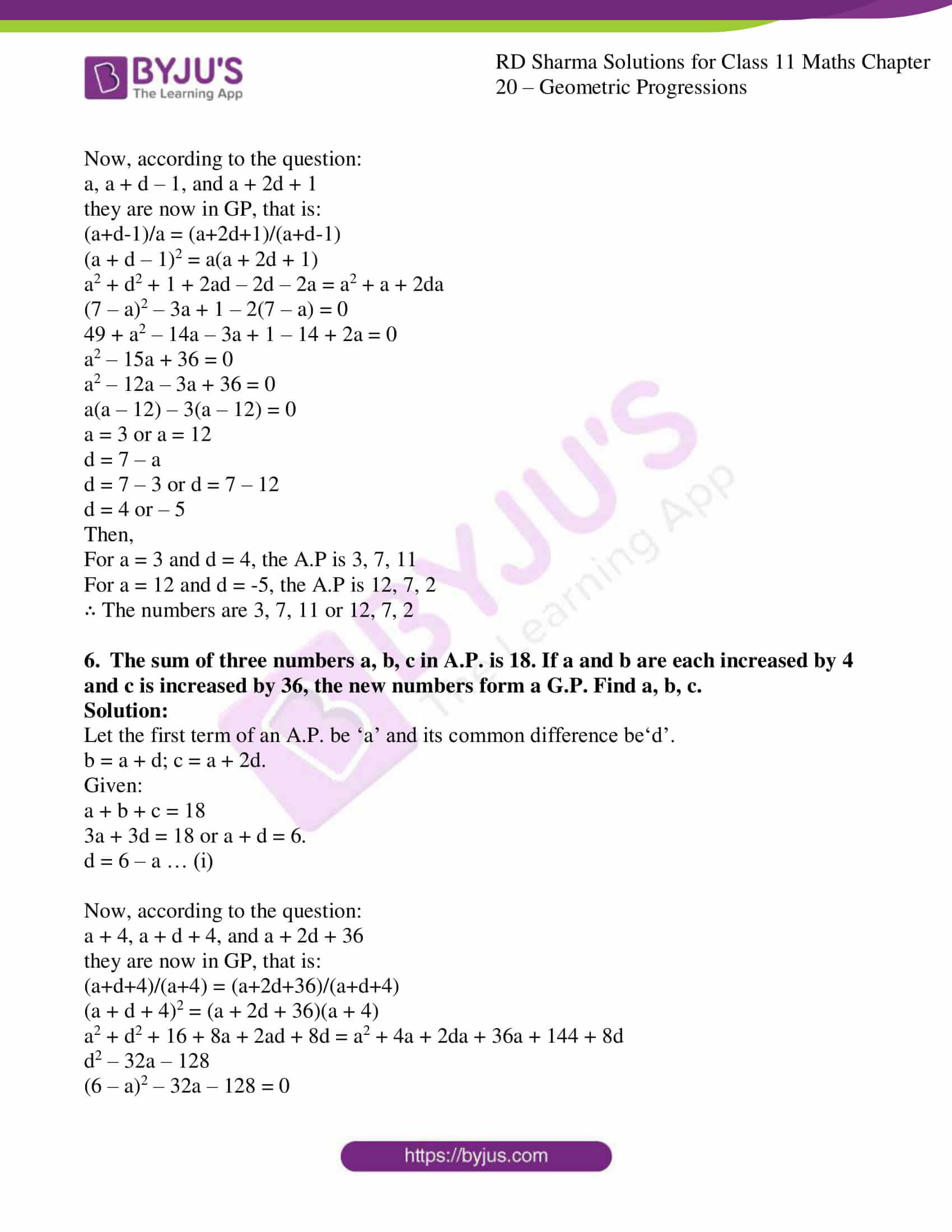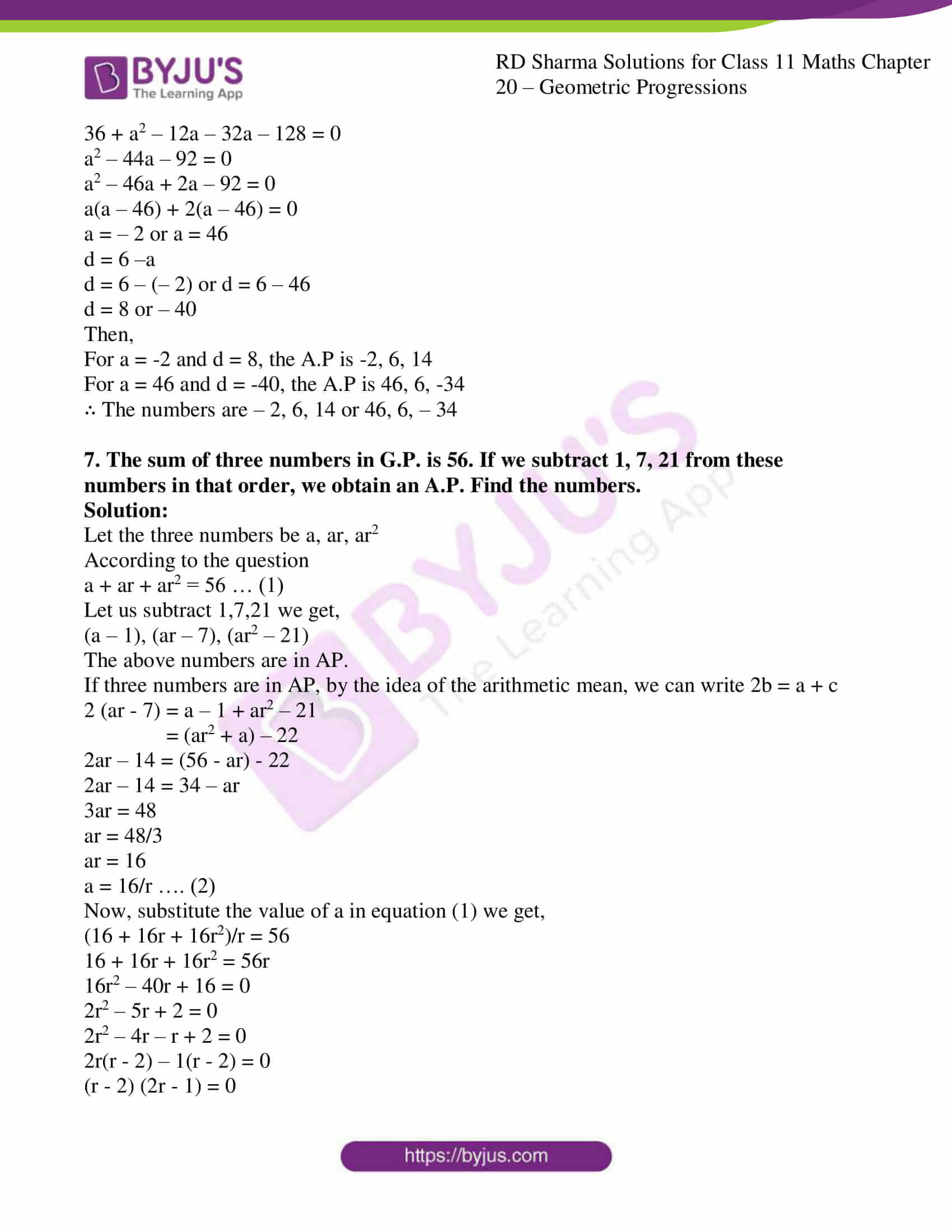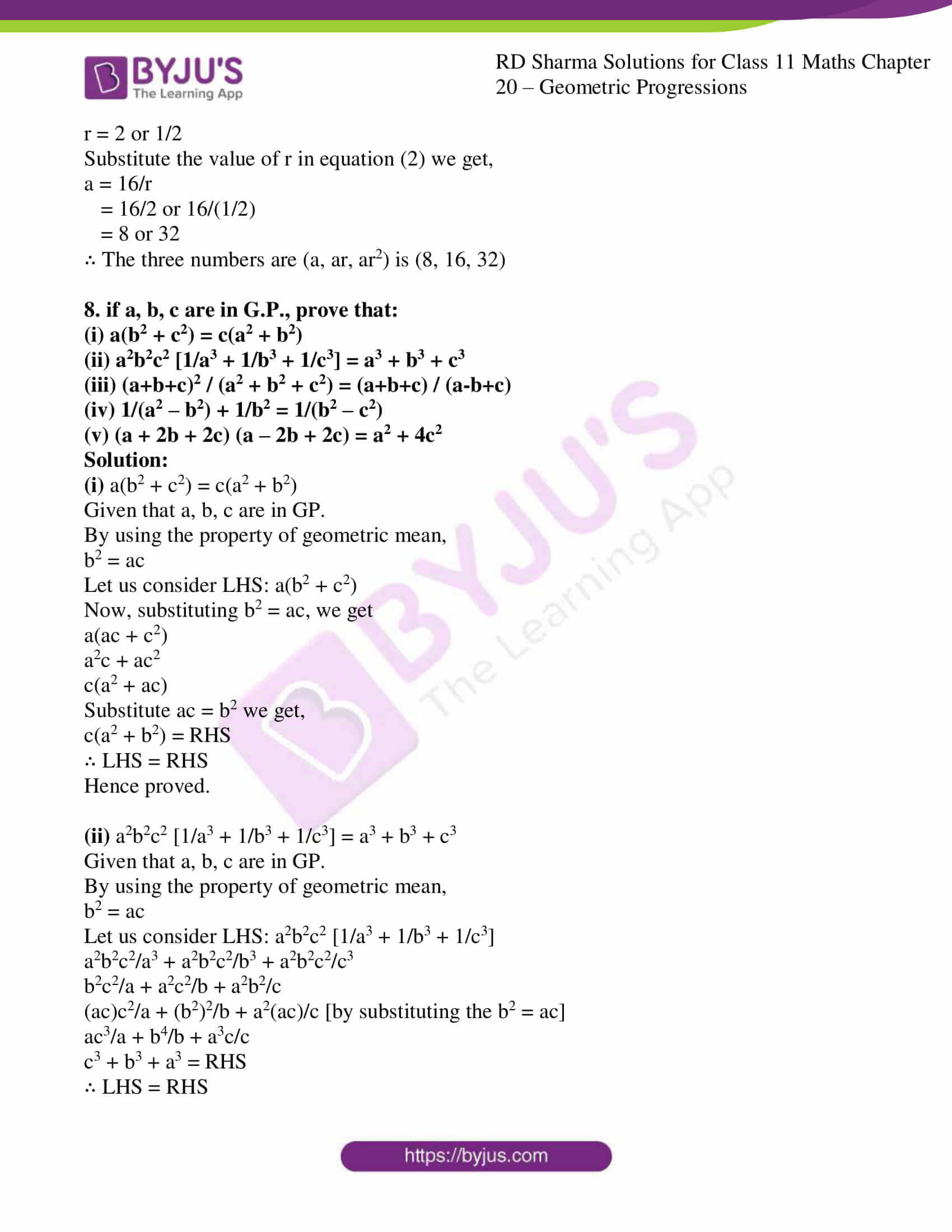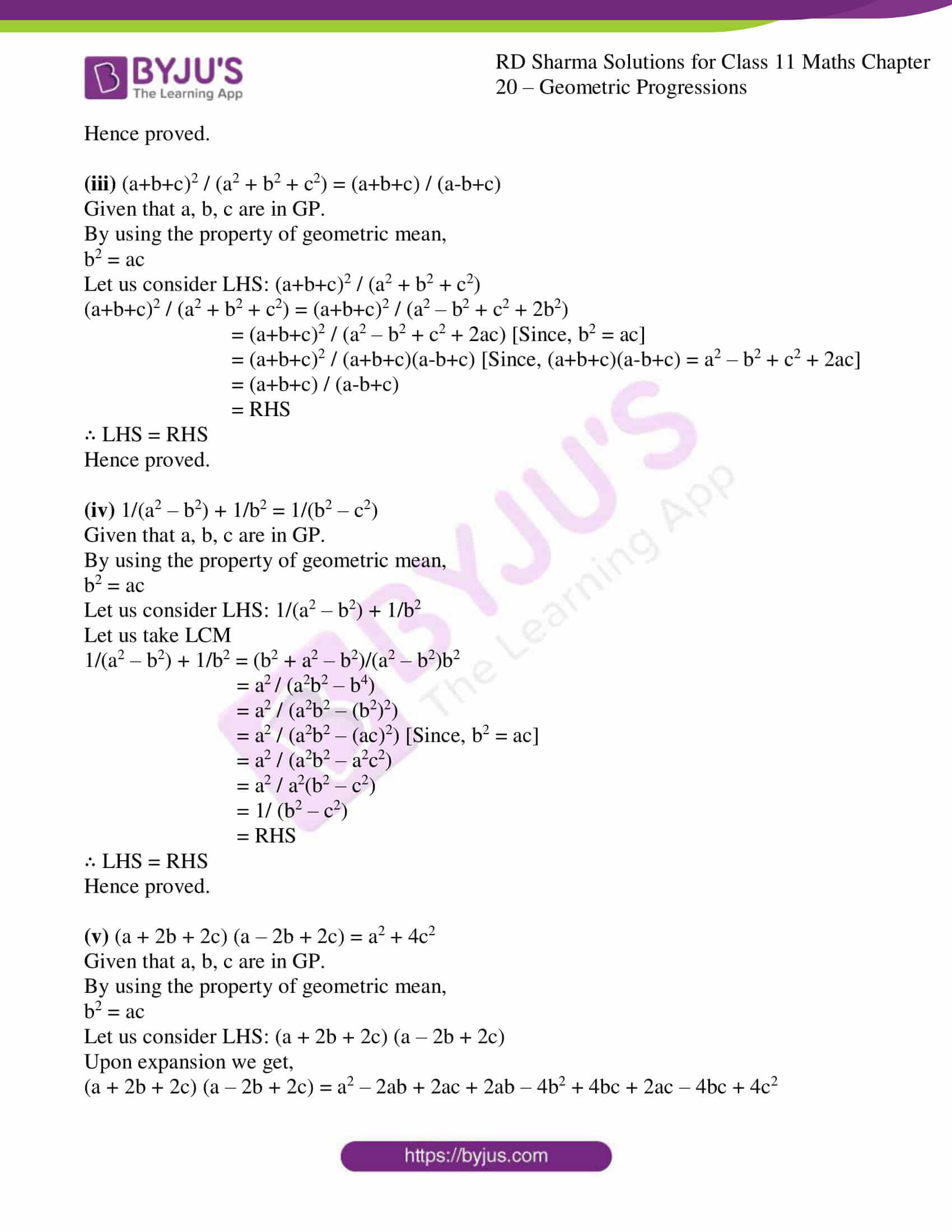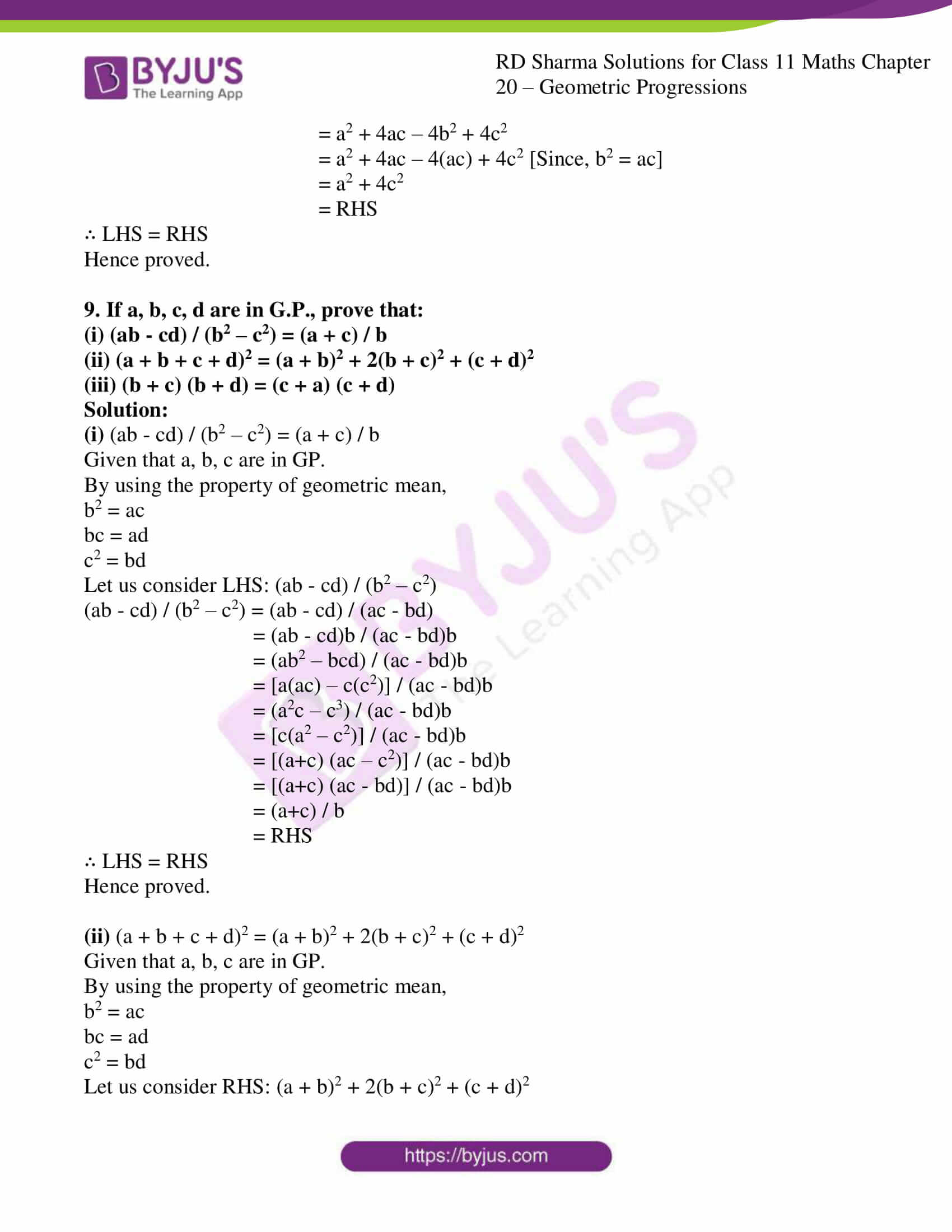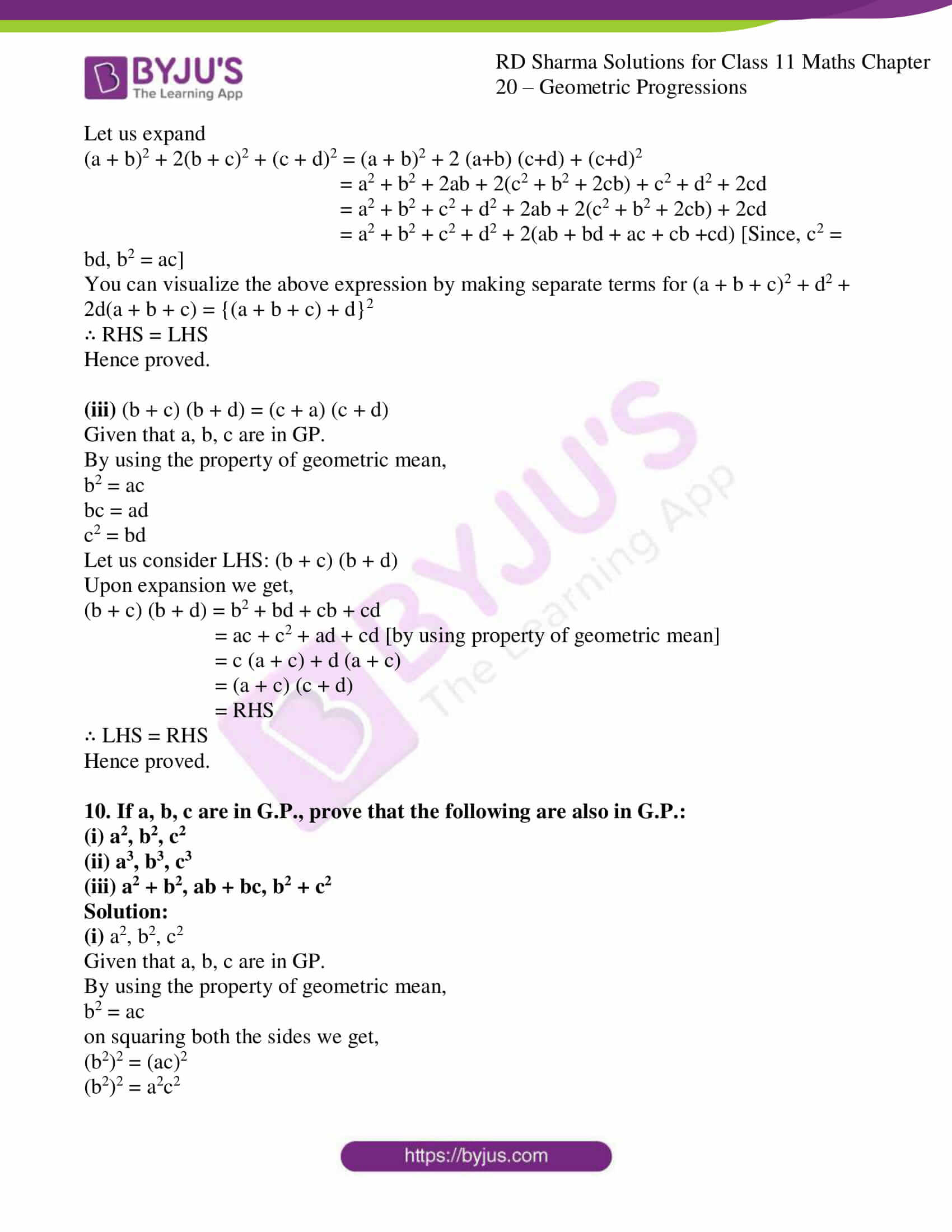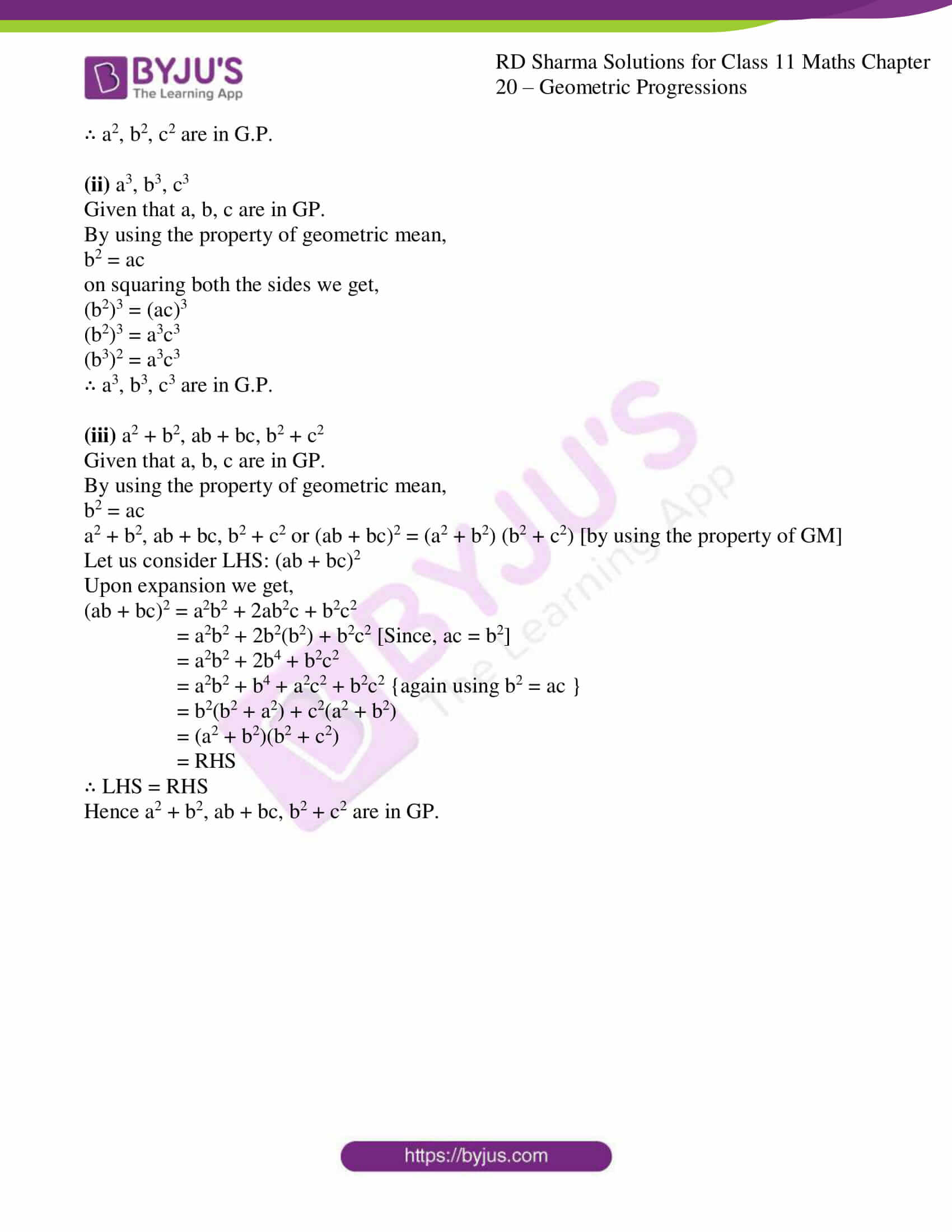### Also, access other exercises of RD Sharma Solutions for Class 11 Maths Chapter 20 – Geometric Progression

Exercise 20.1 Solutions

Exercise 20.2 Solutions

Exercise 20.3 Solutions

Exercise 20.4 Solutions

Exercise 20.6 Solutions

### Access answers to RD Sharma Solutions for Class 11 Maths Exercise 20.5 Chapter 20 – Geometric Progression

1. If a, b, c are in G.P., prove that log a, log b, log c are in A.P.

Solution:

It is given that a, b and c are in G.P.

b2 = ac {using property of geometric mean}

(b2)n = (ac)n

b2n = an cn

Now, apply log on both the sides we get,

log b2n = log (an cn)

log (bn)2 = log an + log cn

2 log bn = log an + log cn

∴ log an, log bn, log cn are in A.P

2. If a, b, c are in G.P., prove that 1/loga m , 1/logb m, 1/logc m are in A.P.

Solution:

Given:

a, b and c are in GP

b2 = ac {property of geometric mean}

Apply log on both sides with base m

logm b2 = logm ac

logm b2 = logm a + logm c {using property of log}

2logm b = logm a + logm c

2/logb m = 1/loga m + 1/logc m

∴ 1/loga m , 1/logb m, 1/logc m are in A.P.

3. Find k such that k + 9, k – 6 and 4 form three consecutive terms of a G.P.

Solution:

Let a = k + 9; b = k−6; and c = 4;

We know that a, b and c are in GP, then

b2 = ac {using property of geometric mean}

(k − 6)2 = 4(k + 9)

k2 – 12k + 36 = 4k + 36

k2 – 16k = 0

k = 0 or k = 16

4. Three numbers are in A.P., and their sum is 15. If 1, 3, 9 be added to them respectively, they from a G.P. find the numbers.

Solution:

Let the first term of an A.P. be ‘a’ and its common difference be‘d’.

a1 + a2 + a3 = 15

Where, the three number are: a, a + d, and a + 2d

So,

a + a + d + a + 2d = 15

3a + 3d = 15 or a + d = 5

d = 5 – a … (i)

Now, according to the question:

a + 1, a + d + 3, and a + 2d + 9

they are in GP, that is:

(a+d+3)/(a+1) = (a+2d+9)/(a+d+3)

(a + d + 3)2 = (a + 2d + 9) (a + 1)

a2 + d2 + 9 + 2ad + 6d + 6a = a2 + a + 2da + 2d + 9a + 9

(5 – a)2 – 4a + 4(5 – a) = 0

25 + a2 – 10a – 4a + 20 – 4a = 0

a2 – 18a + 45 = 0

a2 – 15a – 3a + 45 = 0

a(a – 15) – 3(a – 15) = 0

a = 3 or a = 15

d = 5 – a

d = 5 – 3 or d = 5 – 15

d = 2 or – 10

Then,

For a = 3 and d = 2, the A.P is 3, 5, 7

For a = 15 and d = -10, the A.P is 15, 5, -5

∴ The numbers are 3, 5, 7 or 15, 5, – 5

5. The sum of three numbers which are consecutive terms of an A.P. is 21. If the second number is reduced by 1 and the third is increased by 1, we obtain three consecutive terms of a G.P. Find the numbers.

Solution:

Let the first term of an A.P. be ‘a’ and its common difference be‘d’.

a1 + a2 + a3 = 21

Where, the three number are: a, a + d, and a + 2d

So,

3a + 3d = 21 or

a + d = 7.

d = 7 – a …. (i)

Now, according to the question:

a, a + d – 1, and a + 2d + 1

they are now in GP, that is:

(a+d-1)/a = (a+2d+1)/(a+d-1)

(a + d – 1)2 = a(a + 2d + 1)

a2 + d2 + 1 + 2ad – 2d – 2a = a2 + a + 2da

(7 – a)2 – 3a + 1 – 2(7 – a) = 0

49 + a2 – 14a – 3a + 1 – 14 + 2a = 0

a2 – 15a + 36 = 0

a2 – 12a – 3a + 36 = 0

a(a – 12) – 3(a – 12) = 0

a = 3 or a = 12

d = 7 – a

d = 7 – 3 or d = 7 – 12

d = 4 or – 5

Then,

For a = 3 and d = 4, the A.P is 3, 7, 11

For a = 12 and d = -5, the A.P is 12, 7, 2

∴ The numbers are 3, 7, 11 or 12, 7, 2

6. The sum of three numbers a, b, c in A.P. is 18. If a and b are each increased by 4 and c is increased by 36, the new numbers form a G.P. Find a, b, c.

Solution:

Let the first term of an A.P. be ‘a’ and its common difference be‘d’.

b = a + d; c = a + 2d.

Given:

a + b + c = 18

3a + 3d = 18 or a + d = 6.

d = 6 – a … (i)

Now, according to the question:

a + 4, a + d + 4, and a + 2d + 36

they are now in GP, that is:

(a+d+4)/(a+4) = (a+2d+36)/(a+d+4)

(a + d + 4)2 = (a + 2d + 36)(a + 4)

a2 + d2 + 16 + 8a + 2ad + 8d = a2 + 4a + 2da + 36a + 144 + 8d

d2 – 32a – 128

(6 – a)2 – 32a – 128 = 0

36 + a2 – 12a – 32a – 128 = 0

a2 – 44a – 92 = 0

a2 – 46a + 2a – 92 = 0

a(a – 46) + 2(a – 46) = 0

a = – 2 or a = 46

d = 6 –a

d = 6 – (– 2) or d = 6 – 46

d = 8 or – 40

Then,

For a = -2 and d = 8, the A.P is -2, 6, 14

For a = 46 and d = -40, the A.P is 46, 6, -34

∴ The numbers are – 2, 6, 14 or 46, 6, – 34

7. The sum of three numbers in G.P. is 56. If we subtract 1, 7, 21 from these numbers in that order, we obtain an A.P. Find the numbers.

Solution:

Let the three numbers be a, ar, ar2

According to the question

a + ar + ar2 = 56 … (1)

Let us subtract 1,7,21 we get,

(a – 1), (ar – 7), (ar2 – 21)

The above numbers are in AP.

If three numbers are in AP, by the idea of the arithmetic mean, we can write 2b = a + c

2 (ar – 7) = a – 1 + ar2 – 21

= (ar2 + a) – 22

2ar – 14 = (56 – ar) – 22

2ar – 14 = 34 – ar

3ar = 48

ar = 48/3

ar = 16

a = 16/r …. (2)

Now, substitute the value of a in equation (1) we get,

(16 + 16r + 16r2)/r = 56

16 + 16r + 16r2 = 56r

16r2 – 40r + 16 = 0

2r2 – 5r + 2 = 0

2r2 – 4r – r + 2 = 0

2r(r – 2) – 1(r – 2) = 0

(r – 2) (2r – 1) = 0

r = 2 or 1/2

Substitute the value of r in equation (2) we get,

a = 16/r

= 16/2 or 16/(1/2)

= 8 or 32

∴ The three numbers are (a, ar, ar2) is (8, 16, 32)

8. if a, b, c are in G.P., prove that:

(i) a(b2 + c2) = c(a2 + b2)

(ii) a2b2c2 [1/a3 + 1/b3 + 1/c3] = a3 + b3 + c3

(iii) (a+b+c)2 / (a2 + b2 + c2) = (a+b+c) / (a-b+c)

(iv) 1/(a2 – b2) + 1/b2 = 1/(b2 – c2)

(v) (a + 2b + 2c) (a – 2b + 2c) = a2 + 4c2

Solution:

(i) a(b2 + c2) = c(a2 + b2)

Given that a, b, c are in GP.

By using the property of geometric mean,

b2 = ac

Let us consider LHS: a(b2 + c2)

Now, substituting b2 = ac, we get

a(ac + c2)

a2c + ac2

c(a2 + ac)

Substitute ac = b2 we get,

c(a2 + b2) = RHS

∴ LHS = RHS

Hence proved.

(ii) a2b2c2 [1/a3 + 1/b3 + 1/c3] = a3 + b3 + c3

Given that a, b, c are in GP.

By using the property of geometric mean,

b2 = ac

Let us consider LHS: a2b2c2 [1/a3 + 1/b3 + 1/c3]

a2b2c2/a3 + a2b2c2/b3 + a2b2c2/c3

b2c2/a + a2c2/b + a2b2/c

(ac)c2/a + (b2)2/b + a2(ac)/c [by substituting the b2 = ac]

ac3/a + b4/b + a3c/c

c3 + b3 + a3 = RHS

∴ LHS = RHS

Hence proved.

(iii) (a+b+c)2 / (a2 + b2 + c2) = (a+b+c) / (a-b+c)

Given that a, b, c are in GP.

By using the property of geometric mean,

b2 = ac

Let us consider LHS: (a+b+c)2 / (a2 + b2 + c2)

(a+b+c)2 / (a2 + b2 + c2) = (a+b+c)2 / (a2 – b2 + c2 + 2b2)

= (a+b+c)2 / (a2 – b2 + c2 + 2ac) [Since, b2 = ac]

= (a+b+c)2 / (a+b+c)(a-b+c) [Since, (a+b+c)(a-b+c) = a2 – b2 + c2 + 2ac]

= (a+b+c) / (a-b+c)

= RHS

∴ LHS = RHS

Hence proved.

(iv) 1/(a2 – b2) + 1/b2 = 1/(b2 – c2)

Given that a, b, c are in GP.

By using the property of geometric mean,

b2 = ac

Let us consider LHS: 1/(a2 – b2) + 1/b2

Let us take LCM

1/(a2 – b2) + 1/b2 = (b2 + a2 – b2)/(a2 – b2)b2

= a2 / (a2b2 – b4)

= a2 / (a2b2 – (b2)2)

= a2 / (a2b2 – (ac)2) [Since, b2 = ac]

= a2 / (a2b2 – a2c2)

= a2 / a2(b2 – c2)

= 1/ (b2 – c2)

= RHS

∴ LHS = RHS

Hence proved.

(v) (a + 2b + 2c) (a – 2b + 2c) = a2 + 4c2

Given that a, b, c are in GP.

By using the property of geometric mean,

b2 = ac

Let us consider LHS: (a + 2b + 2c) (a – 2b + 2c)

Upon expansion we get,

(a + 2b + 2c) (a – 2b + 2c) = a2 – 2ab + 2ac + 2ab – 4b2 + 4bc + 2ac – 4bc + 4c2

= a2 + 4ac – 4b2 + 4c2

= a2 + 4ac – 4(ac) + 4c2 [Since, b2 = ac]

= a2 + 4c2

= RHS

∴ LHS = RHS

Hence proved.

9. If a, b, c, d are in G.P., prove that:

(i) (ab – cd) / (b2 – c2) = (a + c) / b

(ii) (a + b + c + d)2 = (a + b)2 + 2(b + c)2 + (c + d)2

(iii) (b + c) (b + d) = (c + a) (c + d)

Solution:

(i) (ab – cd) / (b2 – c2) = (a + c) / b

Given that a, b, c are in GP.

By using the property of geometric mean,

b2 = ac

c2 = bd

Let us consider LHS: (ab – cd) / (b2 – c2)

(ab – cd) / (b2 – c2) = (ab – cd) / (ac – bd)

= (ab – cd)b / (ac – bd)b

= (ab2 – bcd) / (ac – bd)b

= [a(ac) – c(c2)] / (ac – bd)b

= (a2c – c3) / (ac – bd)b

= [c(a2 – c2)] / (ac – bd)b

= [(a+c) (ac – c2)] / (ac – bd)b

= [(a+c) (ac – bd)] / (ac – bd)b

= (a+c) / b

= RHS

∴ LHS = RHS

Hence proved.

(ii) (a + b + c + d)2 = (a + b)2 + 2(b + c)2 + (c + d)2

Given that a, b, c are in GP.

By using the property of geometric mean,

b2 = ac

c2 = bd

Let us consider RHS: (a + b)2 + 2(b + c)2 + (c + d)2

Let us expand

(a + b)2 + 2(b + c)2 + (c + d)2 = (a + b)2 + 2 (a+b) (c+d) + (c+d)2

= a2 + b2 + 2ab + 2(c2 + b2 + 2cb) + c2 + d2 + 2cd

= a2 + b2 + c2 + d2 + 2ab + 2(c2 + b2 + 2cb) + 2cd

= a2 + b2 + c2 + d2 + 2(ab + bd + ac + cb +cd) [Since, c2 = bd, b2 = ac]

You can visualize the above expression by making separate terms for (a + b + c)2 + d2 + 2d(a + b + c) = {(a + b + c) + d}2

∴ RHS = LHS

Hence proved.

(iii) (b + c) (b + d) = (c + a) (c + d)

Given that a, b, c are in GP.

By using the property of geometric mean,

b2 = ac

c2 = bd

Let us consider LHS: (b + c) (b + d)

Upon expansion we get,

(b + c) (b + d) = b2 + bd + cb + cd

= ac + c2 + ad + cd [by using property of geometric mean]

= c (a + c) + d (a + c)

= (a + c) (c + d)

= RHS

∴ LHS = RHS

Hence proved.

10. If a, b, c are in G.P., prove that the following are also in G.P.:
(i) a2, b2, c2

(ii) a3, b3, c3

(iii) a2 + b2, ab + bc, b2 + c2

Solution:

(i) a2, b2, c2

Given that a, b, c are in GP.

By using the property of geometric mean,

b2 = ac

on squaring both the sides we get,

(b2)2 = (ac)2

(b2)2 = a2c2

∴ a2, b2, c2 are in G.P.

(ii) a3, b3, c3

Given that a, b, c are in GP.

By using the property of geometric mean,

b2 = ac

on squaring both the sides we get,

(b2)3 = (ac)3

(b2)3 = a3c3

(b3)2 = a3c3

∴ a3, b3, c3 are in G.P.

(iii) a2 + b2, ab + bc, b2 + c2

Given that a, b, c are in GP.

By using the property of geometric mean,

b2 = ac

a2 + b2, ab + bc, b2 + c2 or (ab + bc)2 = (a2 + b2) (b2 + c2) [by using the property of GM]

Let us consider LHS: (ab + bc)2

Upon expansion we get,

(ab + bc)2 = a2b2 + 2ab2c + b2c2

= a2b2 + 2b2(b2) + b2c2 [Since, ac = b2]

= a2b2 + 2b4 + b2c2

= a2b2 + b4 + a2c2 + b2c2 {again using b2 = ac }

= b2(b2 + a2) + c2(a2 + b2)

= (a2 + b2)(b2 + c2)

= RHS

∴ LHS = RHS

Hence a2 + b2, ab + bc, b2 + c2 are in GP.